Linear Arithmetic Blocks (LAB)

Our preferred model for decimals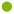Description of LABFeatures of LABHow to make LABUsing LAB as a model of the numberlineUsing the organiserWhy we prefer LAB to MABSample lessonDescription of LABLinear Arithmetic Blocks - our concrete model of choice for teaching decimals!

Numbers are represented by length of pieces of plastic pipe. The longest piece, representing "one" is just over a metre long. This piece is shown to the students first. Discuss cutting into 10 equal pieces. Ask students to use their hands to indicate how long the new piece will be, and what name it should have (a tenth).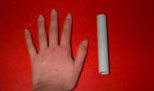Now show the students a tenth piece and discuss cutting it into 10 equal pieces. Again, ask students to predict the length of the new piece and a name (a hundredth).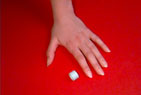Now show the students the hundredth piece and discuss cutting it into 10 equal pieces. Again, ask students to predict the length of the new piece and a name (a thousandth).Now show the students the thousandth piece. We use washers for thousandths because it is too hard to cut such thin pieces of plastic pipe. Then discuss cutting the washer into 10 equal pieces, to make ten-thousandths and beyond to smaller and smaller pieces so that students understand the process that creates the endless base ten chain. In practice, we make nothing smaller than thousandths.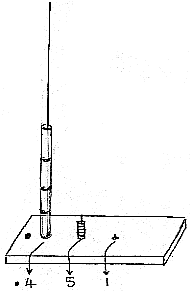LAB pieces can be arranged randomly in piles or in 2 systematic ways. On the right, they are shown on an organiser. The organiser is a wooden stand with three dowel rods to hold nine tenths, hundredths and thousandths. This is very useful to demonstrate trading in the addition and subtraction algorithms. In the 3 photos below, the pieces are laid end to end to confirm that:10 tenths have the same length as the one piece10 hundredths have the same length as the tenth piece10 thousandths have the same length as the hundredth pieceA larger photo of the organiser in use.Features of LAB as a modelUses the physical quantity of length to represent the size of a number.Represents size of number from the digits in the numeral very well.Represents base 10 properties (bundling and column overflow) very well.Useful from ones to thousandths and can be mentally extended easily in both directions. (This is an important discussion to have with students!)The "endless base ten chain" multiplicative relations between the values of places are shown reasonably well.Demonstrates addition and subtraction algorithms well.Demonstrates multiplication and division of a decimal by a small whole number or by a power of ten well.Only some divisions by a decimal can be shown well (selected quotitions). This is a general limitation of concrete models.Multiplication by a decimal cannot be easily demonstrated with LAB.LAB represents numbers by the quantity of length, not by units of length such as millimetres. This is an important distinction because using units of length may perpetuate misconceptions that the decimal point simply separates one whole-number quantity (the number of metres) from another (the number of millimetres). It happens that the unit piece is approximately one metre long, but this is only a consequence of the size of suitable materials.How to make LAB

 Linear Arithmetic Blocks (LAB) can be made at home or at school from ordinary washers and PVC pipe of a similar diameter. To make LAB, first purchase the washers (thousandths). The next pieces (hundredths) are then made by cutting small lengths of plastic tubing to exactly to the length of 10 washers. Then make tenths (medium size lengths of tubing ) cut to 10 hundredths. Lastly, the one (whole) is cut to 10 tenths and is a rather long piece of tube, probably over a metre long (as the washer may well be more than 1 mm thick).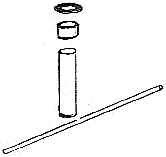A good set for classroom demonstration requires about 7m of 25 mm diameter plastic pipe and contains about 40 thousandths about 30 tenths and hundredths at least two ones. Children's sets can have fewer pieces. The wooden organiser is optional. It is made of 3 rods (tenths, hundredths and thousandths) set into a base of wood. A decimal point can be drawn on the base with texta. The heights of the rods on the organiser are such that only 9 of the pieces can be placed on the relevant rod. It is obviously not practical to make a rod for the ones, as it would be over 9 metres high! (Involve students in this discussion) Approximately 1.5 m of dowel is required and a base measuring approximately 30cm x 15cm x 10cm.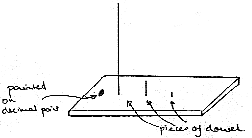Using LAB as a model of the numberline

 In the pictures below, the LAB pieces representing the decimals have been laid linearly instead of on the organiser. This allows us to compare the total length of the pieces and hence the size of the decimals they represent.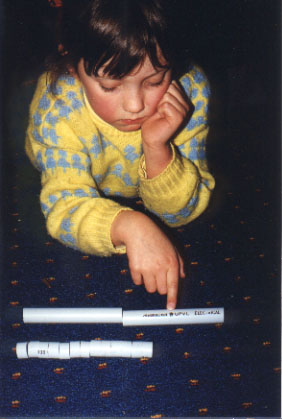LAB clearly shows that 0.2 is larger than 0.13 and not the other way around! Many longer-is-larger children think that 0.13 is larger than 0.2 (as 13 is larger than 2). It also shows other properties clearly including: equivalence of 0.2 and 0.20 (2 tenths and 20 hundredths) equivalence of 0.13 (13 hundredths) with 0.1 + 0.03 (one tenth and 3 hundredths) density of decimal numbers (that there are other decimals between 0.24 and 0.25 or between 0.247 and 0.248 etc)LAB can be used to round decimal numbers. For example, to round 0.27 to the nearest tenth, make several numbers using only the tenths pieces (1 tenth, 2 tenths, 3 tenths, 4 tenths...) and compare. Children can see that the number 0.27 is between 2 tenths and 3 tenths and closer to 0.3. Many children and even some adults are confused by numberlines. Building a number line is the ideal way to help students understand the ideas involved.Using the organiser

 The organiser serves a similar purpose to the place value chart often used with MAB, holding pieces of the same size together and representing the left-right spatial arrangement of decimal numeration. (Beware - you must be looking from the front!) It also if there are ten or more pieces of any one size, they will not fit onto the appropriate rod and so ten of them must be exchanged for a single piece of the next highest value. This forces the trading required in the algorithms.Overuse of the organiser or place value chart may reduce the LAB or MAB model (in the children's minds) to that of an abacus; where it is the position of the beads only which represents the size of the number. To ensure that students do not become reliant on the organiser (or place value chart), make sure that students:appreciate that the size of the number represented does depend on the physical length of the blocks and not their arrangement on a chart, andare able to move the blocks onto the organiser (by themselves) to assist in reading off the symbolic representation.Why we prefer LAB to MAB

Our research has demonstrated that LAB has a number of advantages over MAB.

Our published research paper (Stacey et al, 2001a) compares the two materials on the basis of epistemic fidelity (how true the model is to the mathematical principles involved) and accessibility for students. Two teaching experiments involving 30 matched students indicated that LAB is considerably more accessible for students. There are three reasons for this:students get confused with MAB simply because it has been used before with the "mini" representing one (see above);LAB models number with length whereas MAB models number with volume and many students in upper primary do not yet have a strong grasp of volume;the various pieces of MAB seem to be of different dimensions (1-D, 2-D, 3-D ) and this makes generalizing to more place value columns difficult.

Use of LAB was associated with more active engagement by students and deeper discussion. Epistemic fidelity is critical to facilitate teaching with the models, but we attribute the enhanced classroom environment to the greater accessibility of the LAB material.

Both models have excellent epistemic fidelity, so that they both show how the size of numbers depends on the digits and the place value columns and they both can be used to demonstrate the operations. However, a significant difference between the two models is that LAB, with pieces laid end to end, has structural similarity to the number line, and is thus better able than MAB to model number density (the property that between any two decimals, a third decimal can always be inserted). LAB is therefore better able to demonstrate the principles of rounding. The limitations of MAB in regard to the continuous properties of decimals were noted by Hiebert, Wearne and Taber (1991). Following instruction with MAB, Year 4 students' performance on discrete-context tasks (e.g. writing the number represented by a picture of MAB; choosing the larger of two decimals) improved. However their performance on continuous-representation tasks (e.g. shading 2.6 of a continuous quantity; finding a number between two decimals) did not. Thus MAB appeared to support understandings of decimals as discrete quantities but not as continuous quantities.

TopSample lesson plan

LAB was first shown to us by Heather McCarthy, a Melbourne teacher. Heather had seen it at an in-service day, but we cannot trace the source. Heather's worksheets.

Top